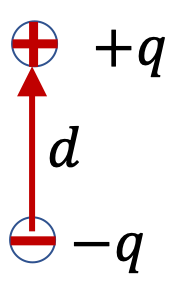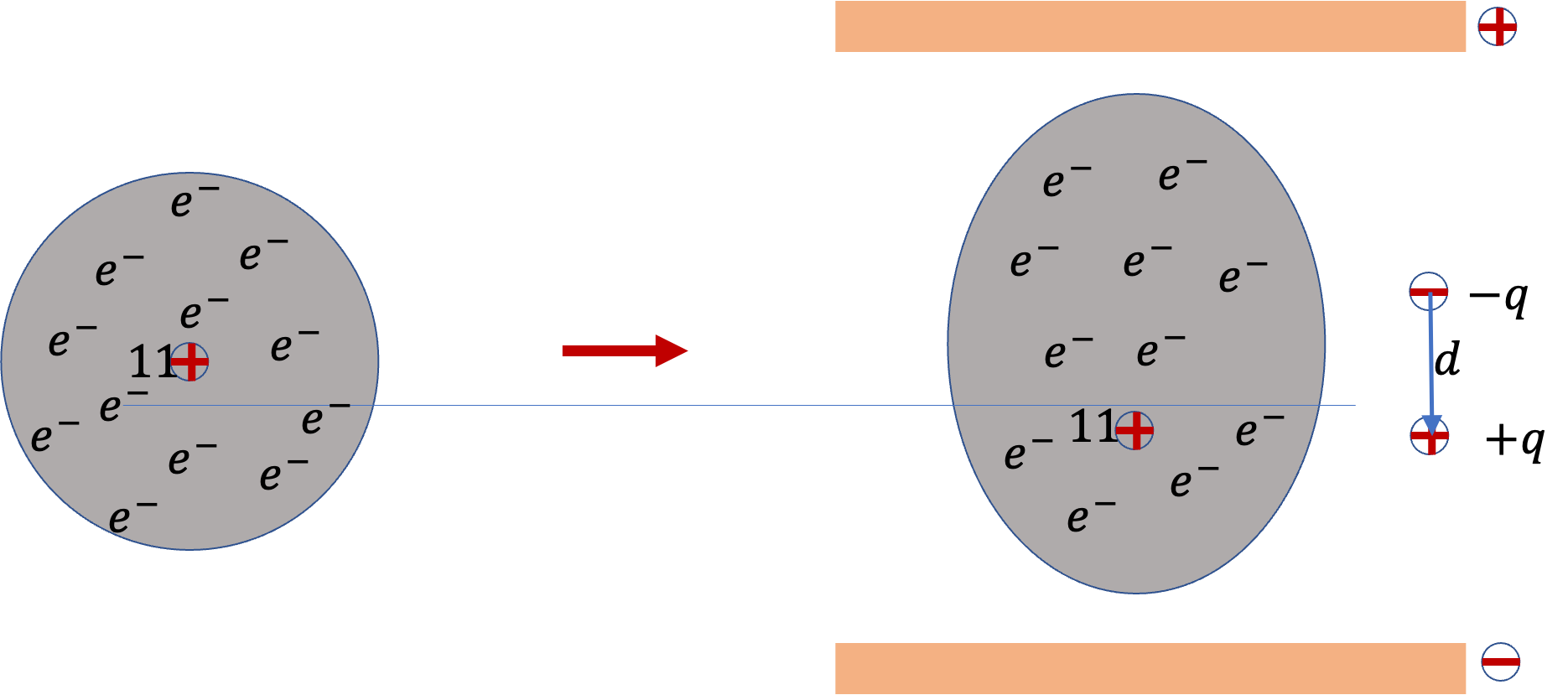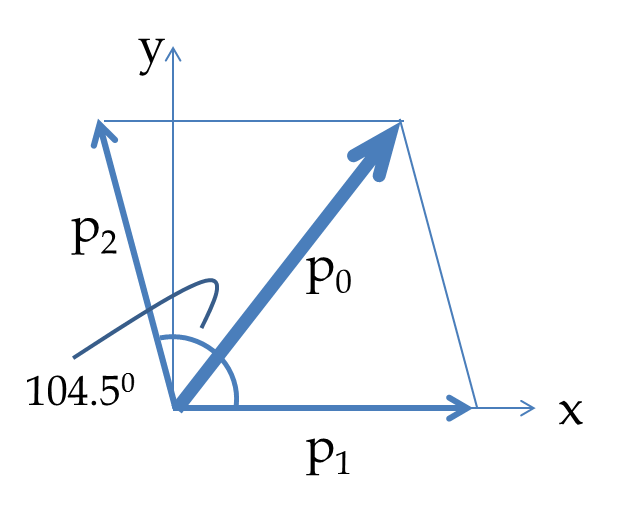## Section33.1Electric Dipoles

### Subsection33.1.1Electric Dipoles and Electric Dipole Moment

Two equal but opposite charges make up a dipole.

Let charges be denoted by $\pm q$ and let their separation be $d\text{.}$ The product of amount of charge and their separation is called electric dipole moment, which is denoted by letter $p\text{.}$\begin{equation} p = q\,d.\label{eq-electric-dipole-moment-definition}\tag{33.1.1} \end{equation}

We will also make use of dipole moment vector, $\vec p\text{,}$ whose magnitude is $qd\text{,}$ and direction is the direction from the negative charge towards the positive charge.

### Subsection33.1.2Induced Dipoles

If you place an atom in an electric field, its electrons will be pulled one way and the protons will be pulled the other way which distorts the electron cloud around nucleus as illustrated in Figure 33.1.1. As a result, one side of the atom will become more negative and the other side equally positive. This results in atom becoming polarized while in this field. This type of dipole is called an induced dipole.Figure 33.1.1. Induced polarization of a spherically symmetric sodium ($\text{Na}$) atom in an external electric field. (a) Symmetric electron cloud when there is no electric field, (b) Asymmetric electron cloud in the presence of an external electric field, created here by charges on two metal plates. The summary diagram in (b) shows effectively, two point charges $\pm q$ separated by a distance $d$ with a dipole moment of $p=qd\text{.}$

It would not surprise you that induced dipole moment will increase with increasing electric field. If electric field, $E\text{,}$ is not too great, the dipole moment induced is proportional to the electric field as shown in Figure 33.1.2. We say that we have a linear dielectric.

We express the linear relation as follows, where constant of proportionality $\alpha$ is called the atomic polarizability.

\begin{equation*} p_{\text{ind}} = \alpha\, E. \end{equation*}

Experimentally determined atomic polarizabilities of several atoms are given in Table 33.1.3 . Notice that the atomic polarizability decreases across a period of the periodic table as the electrons of the atoms become more tightly bound to the nuclei. However, as you go down in a group to larger atoms, e.g. H, Li, Na, K, ... etc, the valence electrons are further out from the nucleus and less tightly bound, and therefore more easily polarizable as indicated by an increase in the atomic polarizability.

### Subsection33.1.3Permanent Dipole Moments

When dissimilar atoms bond in a molecule, a separation of charge results due to the bonding electrons being attracted more to the nucleus of one atom than to the other. This separation of charges gives rise to permanent dipole moments. This dipole moment would be present even in the absence of any applied electric field.

For instance, in a water molecule, electrons are attracted more to the oxygen atom than to the hydrogen atoms making the oxygen atom negatively charged and the hydrogen atoms positively charged as shown in Figure 33.1.4.

The separation of charges between oxygen and hydrogen atoms results in a permanent dipole moment along each O-H bond. Since the angle between the two O$-$H bonds in water is $104.5^{\circ}\text{,}$ the vector addition of the dipole moment vectors along the O$-$H bonds gives a net dipole moment vector of magnitude $6.1 \times 10^{-30}$ C.m pointed towards the middle of the H$-$O$-$H angle. This is a very large dipole moment compared to the dipole moments of other molecules and is responsible for many of the useful properties of water.

Of course, when you place a molecule with a permanent dipole moment in an external electric field, you may polarize the molecule even more or reduce the polarization. This may give rise to a net dipole moment which may be higher or lower than the permanent dipole moment. We will see below that external field may also apply a torque and rotate the dipole.

The dipole moment of a carbon atom in an external electric field has magnitude $2.5 \times 10^{-33}\text{ C.m}\text{.}$ What is the magnitude of the applied electric field?

Data: $\alpha_C = 2\times 10^{-40}\:\text{C.m}^2/\text{V}\text{.}$

Hint

Use definition.

$1.25\times 10^7\text{ N/C}\text{.}$

Solution

Using the formula for induced dipole in an atom, we get

\begin{align*} E \amp = \dfrac{p}{\alpha}\\ \amp = \dfrac{2.5\times 10^{-33}\:\text{C.m}}{2\times 10^{-40}\:\text{C.m}^2/\text{V}}\\ \amp = 1.25\times 10^{7}\:\text{V/m}. \end{align*}

Evaluate the magnitude of the dipole moment of a sodium atom induced when the atom is between two large parallel aluminum plates separated by $3\text{ mm}$ where a potential difference of $100\text{ V}$ is maintained.

Data: $\alpha_\text{Na} = 27\times 10^{-40}\:\text{C.m}^2/\text{V}\text{.}$

Hint

Use $E=\phi/d$ to get the field.

$9\times 10^{-34}\:\text{C.m}\text{.}$

Solution

The magnitude of the electric field between the plates will be

\begin{equation*} E = \dfrac{V}{d} = \frac{100\:\text{V}}{0.003\:\text{m}} = \dfrac{1}{3}\times 10^{5}\:\text{V/m}. \end{equation*}

Therefore, the dipole moment of sodium atom will be

\begin{align*} p \amp = \alpha E\\ \amp = 27\times 10^{-40}\:\text{C.m}^2/\text{V} \times \dfrac{1}{3}\times 10^{5}\:\text{V/m}\\ \amp = 9\times 10^{-34}\:\text{C.m}. \end{align*}

The water molecule has electric dipole moment of $6.1 \times 10^{-30}$ C.m and the H$-$O$-$H angle is $104.5^{\circ}\text{.}$ (a) What is the dipole moment in each OH bond? (b) If the O$-$H bond distance is $0.0965$ nm, how much net charges reside on hydrogen and oxygen atoms written in terms of charge on one electron?

Hint

Use vector addition of bond dipole moments to get the net dipole moment.

(a) $5.0\times 10^{-10}$ C.m, (b) $0.323\ e\text{.}$

Solution 1 (a)

(a) Let $p$ be the unknown dipole moment of each OH bond. The dipole moment of the water molecule is equal to the vector sum of the dipole moments of the OH bonds which are at an angle of $104.5^{\circ}\text{.}$Let $p$ denote the magnitude of the dipole moment of the OH bond, denoted as $\vec p_1$ and $\vec p_2$ in the figure. Equating the $x$-component of the dipole moment $\vec p_0$ of the water molecule to the sum of the $x$-components of the OH bonds gives

\begin{equation*} p_0\:\cos(\theta/2) = p + p\cos\theta, \end{equation*}

where $\theta = 104.5^{\circ}\text{,}$ the angle between the OH bonds. Solving for $p$ we get

\begin{equation*} p = \left[ \dfrac{\cos(\theta/2)}{1+ \cos\theta} \right] \: p_0. \end{equation*}

Now, putting the numerical values we find the following for the magnitude of the dipole moment of the OH bond.

\begin{equation*} p = \left[ \dfrac{\cos(52.25^{\circ})}{1+ \cos(104.5^{\circ})} \right] \times 6.1 \times 10^{-30}\: \textrm{C.m} = 4.98\times 10^{-30}\: \textrm{C.m}. \end{equation*}
Solution 2 (b)

(b) The dipole moment of OH bond is as a result of the polarization of the bond with a positive charge $q$ on H and equal negative charge $-q$ on O. Let $l$ be the OH bond length, then $p = ql\text{.}$ Therefore, the charges on the two atoms have the following magnitude

\begin{equation*} q = \dfrac{p}{l} = \dfrac{4.98\times 10^{-30}\: \textrm{C.m}}{0.0965\times 10^{-9}\:\textrm{m}} = 5.16\times 10^{-20}\:\textrm{C} = 0.323\:e. \end{equation*}